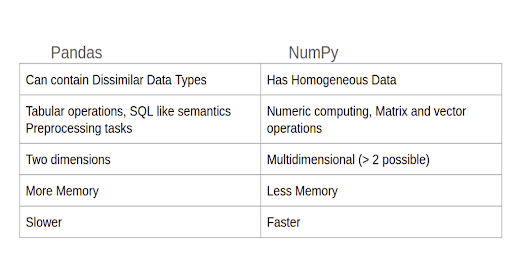# Differences between Pandas and NumPy

Pandas and NumPy are two of the most popular python libraries used for data science applications.

##### What is Numpy?

Numpy is a popular library used for scientfic computing. It has support for multidimensional arrays and mathematical functions that can operate on these arrays.

NumPy arrays are homogeneously typed – which means they hold elements of the same type. NumPy is designed specifically to speed up operations that operate on arrays and is memory efficient compared other heterogeneous data structures commonly used in python.

##### What is Pandas ?

Pandas is built on top of numpy and is used for preprocessing tasks and other analysis tasks in a typical data science pipeline. It is slower than numpy and usually takes more memory, but offers extra convenience to handle tabular data.

Specifically, pandas supports SQL like operations such as querying and joins that makes it useful for wrangling data for preprocessing tasks such as handling missing data, understanding outliers and other common tasks.

##### What are the differences between Pandas vs NumPy?